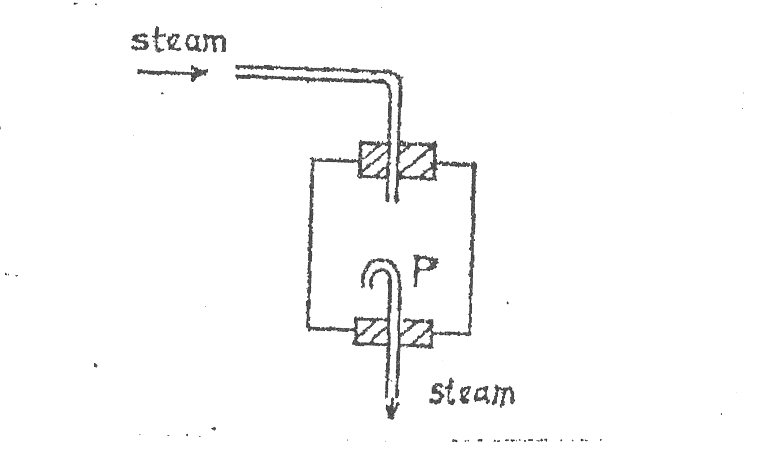Home Technical Mathematics Languages Science Social Science Art Literature Arabic Islamic Studies C.R.K History MusicVisual Art Clothing/Textile Home Management Shorthand
 Physics Paper 2, Nov/Dec. 2009
 Questions: 1 2 3 4 5 6 7 8 9 10 11 12 13 14 15 Main
Weakness/Remedies
Strength

Question 12
Part II:      Candidates were required to answer any five questions from this part.

(a)  (i)         Define latent heat of fusion.
(ii)        DiagramThe diagram above illustrates part of the set-up for determining the specific latent heat of vaporization of water.  State the purpose of the curved tube P.

(b) Explain how pure water can be made to boil at a temperature below 100o C.

(c) The resistance in the element of a platinum resistance thermometer is 9.60 Ω at 0.oC, 12.10 Ω at 100oC and 10.20Ω room temperature.  Calculate the room temperature on the scale of the resistance thermometer.

(d) A body of mass 2 kg at 100 oC is dropped gently into a mixture of ice and water at 0oC.  Calculate the mass of ice that melted at 0oC.

Specific latent heat of fusion of ice = 3.3 x 105 J kg-1
Specific heat capacity of the body = 2.2 x 103 J kg-1 K-1

_____________________________________________________________________________________________________
Observation

Part (a) was well attempted.  However some candidates mistook specific latent heat of fusion for latent heat of fusion.

The purpose of tube P was poorly identified indicating poor knowledge of experiment used for determining the specific latent heat of vaporization of water.

Part (b) was poorly attempted by majority of the responding candidates though many candidates recalled the fact that pure water boil at a temperature below 100oC when its s.v.p is lower than normal atmospheric pressure.   However, majority of the candidates could not explain such using a round bottom flask.  Some even attributed it to impurities rather than reduction in pressure.

Part (c) was well attempted by majority of the responding candidates.

Part (d) was fairly well attempted though some candidates used wrong formula.

The expected answers were as follows:

(a)        (i)         This is the amount of heat required to change a substance from the solid
state to the liquid state without a change in/at constant temperature.
(ii)        It is to ensure that only dry steam is used.

(b)        Pure water boils at a temperature below 100oC when its s.v.p is less than normal atmospheric pressure.

To achieve this:

• Fill a round bottom flask to ¾ of its volume.
• Insert a thermometer through the stopper and heat the flask to about 80oC.
• Invert the flask and place it under flowing tap water so as to reduce the pressure in the flask as a result of condensation of vapour.

(c)         θ     =  Re  ­-  Ro
100       R100  - Ro

θ   =   10.2  -  9.6  x 100
12.1  -  9.6

=   0.6  x 100
2.5

=   24. 0o C

• Heat lost by hot body  =  Heat gained by ice

MbCb Δθ  =  MiLf
2 x 2200 x (100 – 0)  =  Mi  x 330000

Mi  =  2 x 2200 x 100   =  4/3
330000

=  1.33 kg.